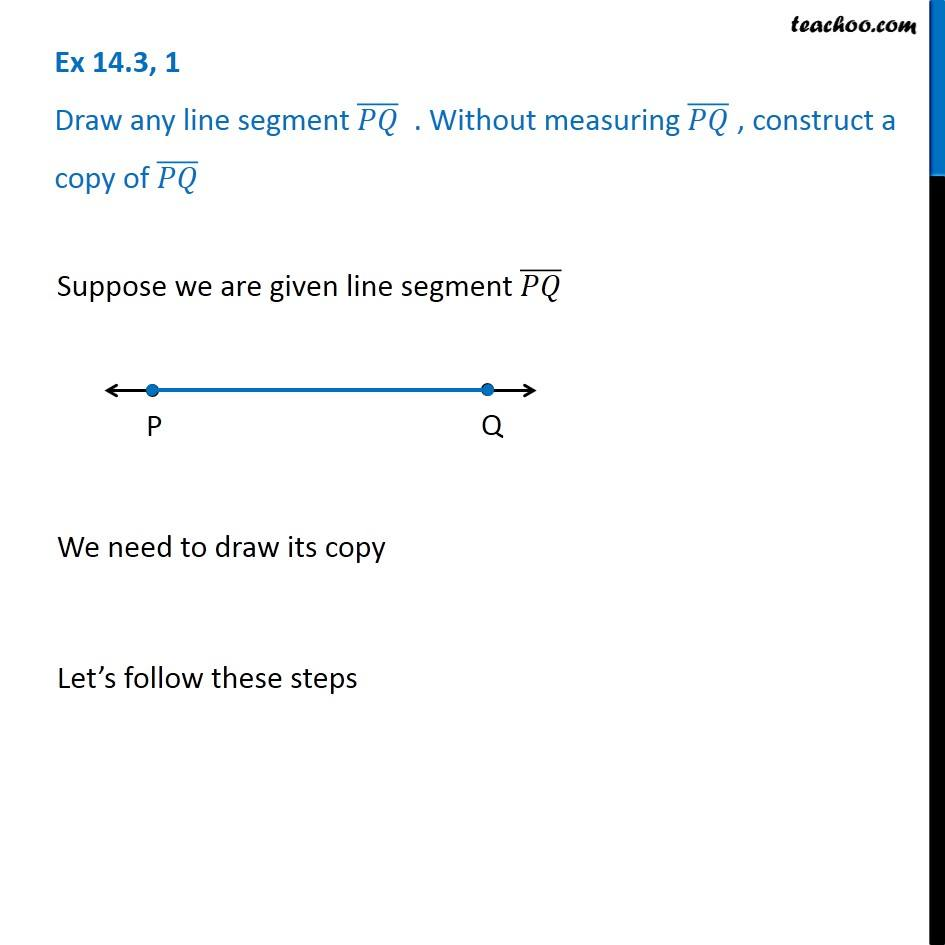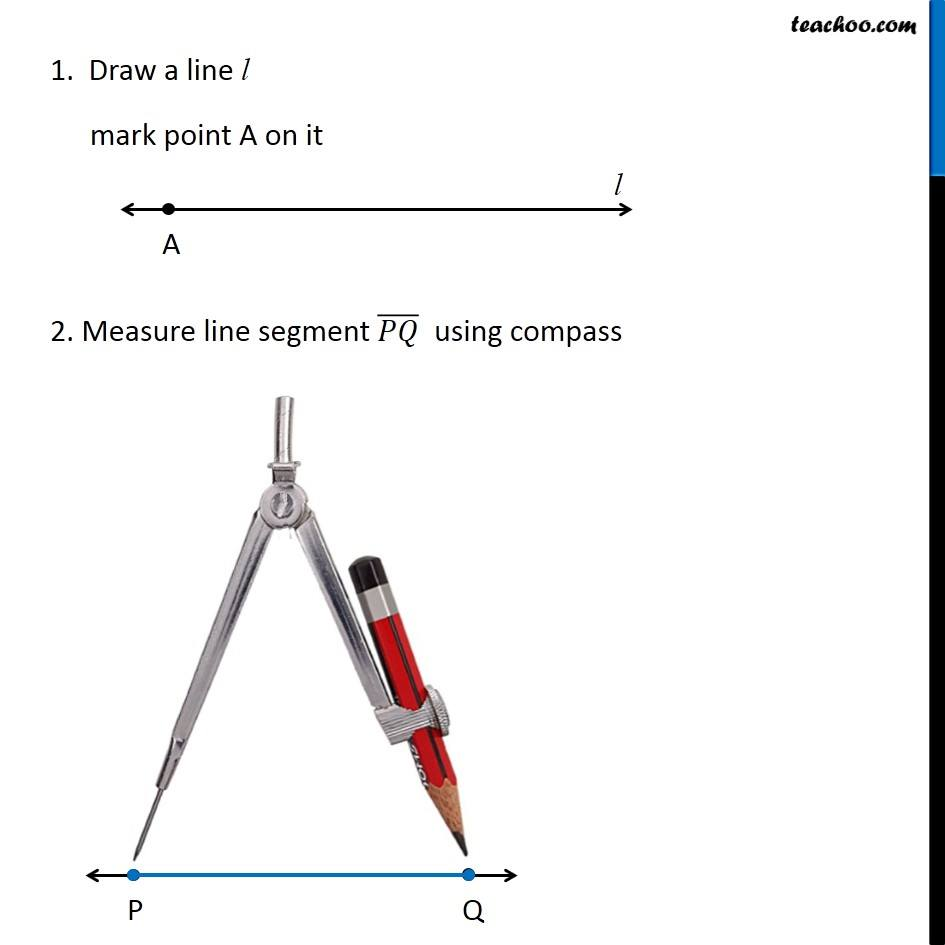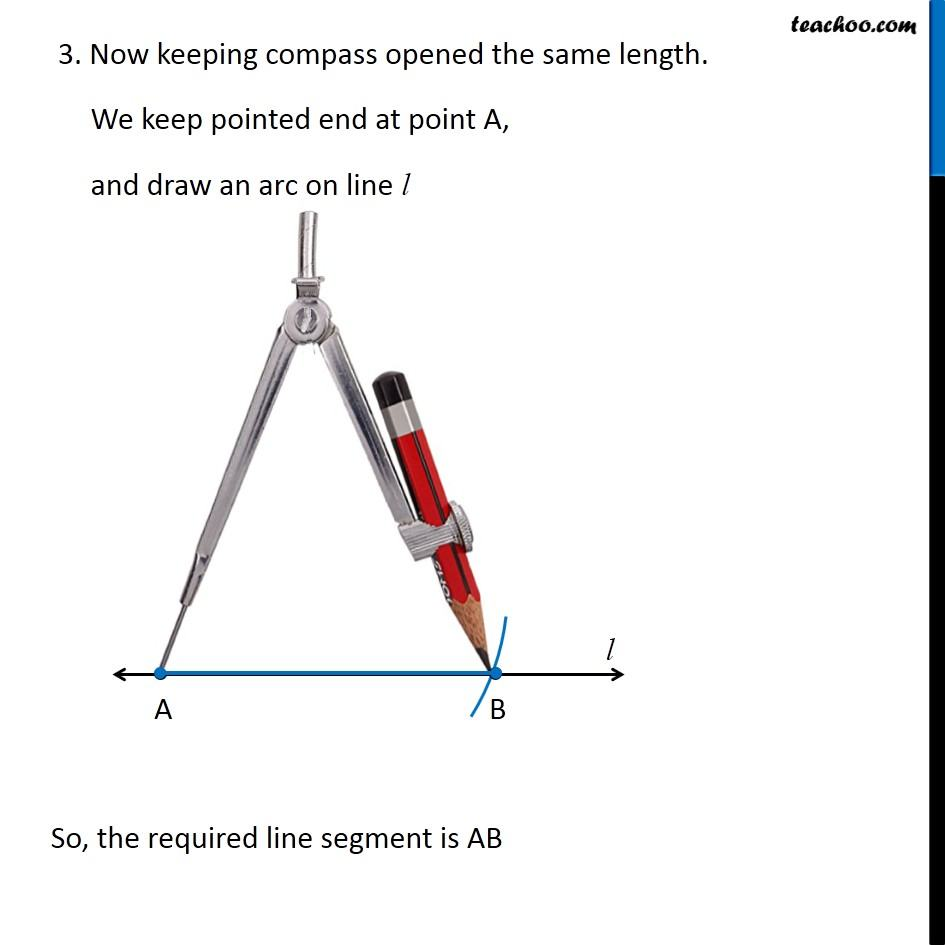1. Chapter 14 Class 6 Practical Geometry
2. Serial order wise
3. Ex 14.3

Transcript

Ex 14.3, 1 Draw any line segment (𝑃𝑄) ̅ . Without measuring (𝑃𝑄) ̅ , construct a copy of (𝑃𝑄) ̅ Suppose we are given line segment (𝑃𝑄) ̅ We need to draw its copy Let’s follow these steps 1. Draw a line l mark point A on it 2. Measure line segment (𝑃𝑄) ̅ using compass 3. Now keeping compass opened the same length. We keep pointed end at point A, and draw an arc on line l So, the required line segment is AB